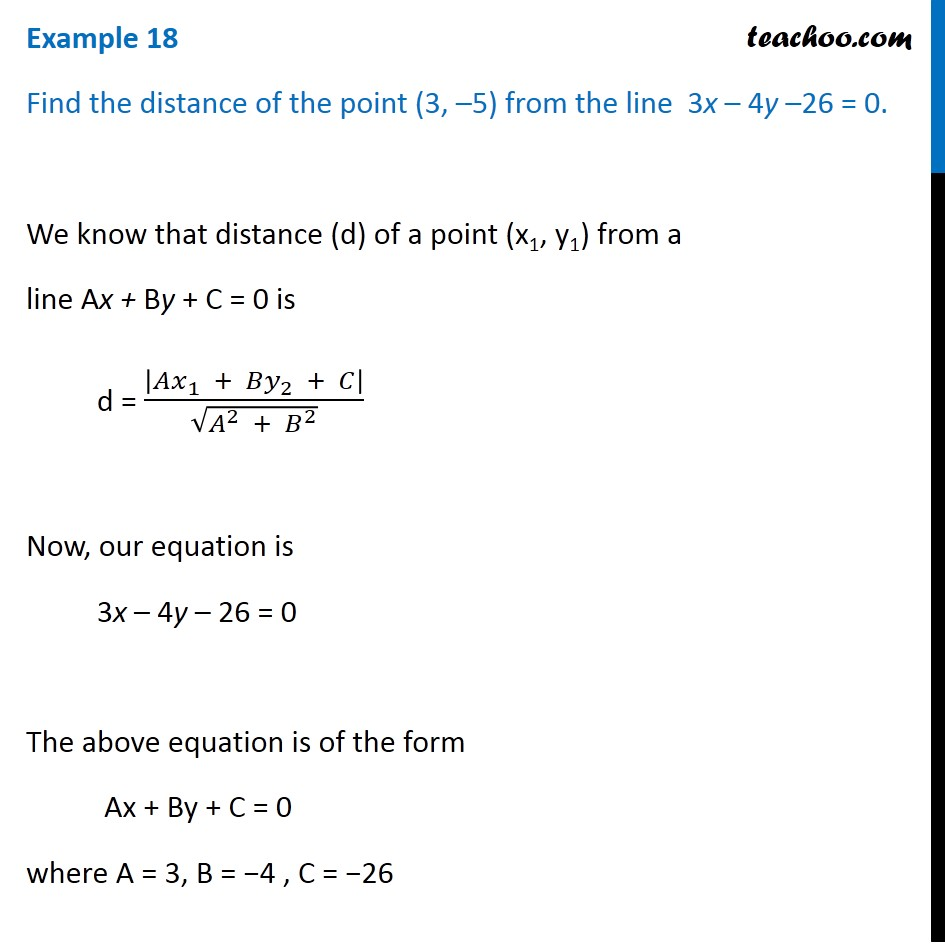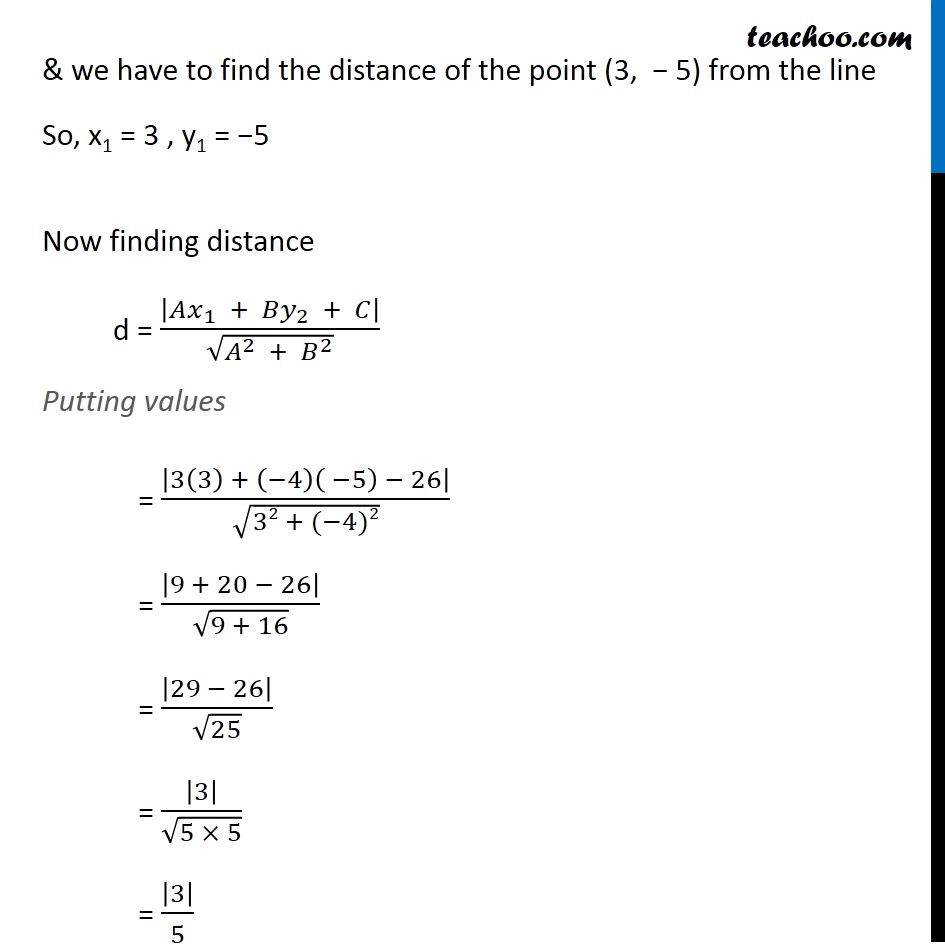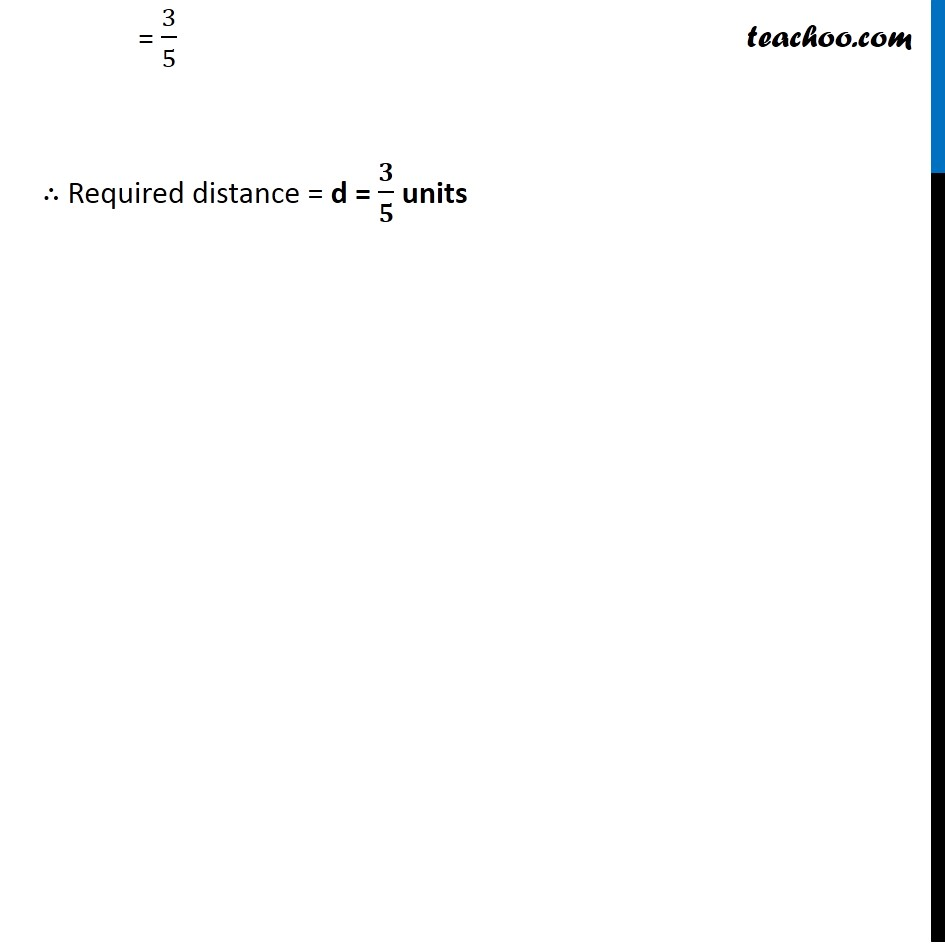Examples

Chapter 9 Class 11 Straight Lines
Serial order wiseLearn in your speed, with individual attention - Teachoo Maths 1-on-1 Class

### Transcript

Example 9 Find the distance of the point (3, –5) from the line 3x – 4y –26 = 0. We know that distance (d) of a point (x1, y1) from a line Ax + By + C = 0 is d = |𝐴𝑥_1 + 〖𝐵𝑦〗_2 + 𝐶|/√(𝐴^2 + 𝐵^2 ) Now, our equation is 3x – 4y – 26 = 0 The above equation is of the form Ax + By + C = 0 where A = 3, B = −4 , C = −26 & we have to find the distance of the point (3, − 5) from the line So, x1 = 3 , y1 = −5 Now finding distance d = |𝐴𝑥_1 + 〖𝐵𝑦〗_2 + 𝐶|/√(𝐴^2 + 𝐵^2 ) Putting values = |3(3) + (−4)( −5) − 26|/√(32 + (−4)2) = |9 + 20 − 26|/√(9 + 16) = |29 − 26|/√25 = |3|/√(5 × 5) = |3|/5 = 3/5 ∴ Required distance = d = 𝟑/𝟓 units